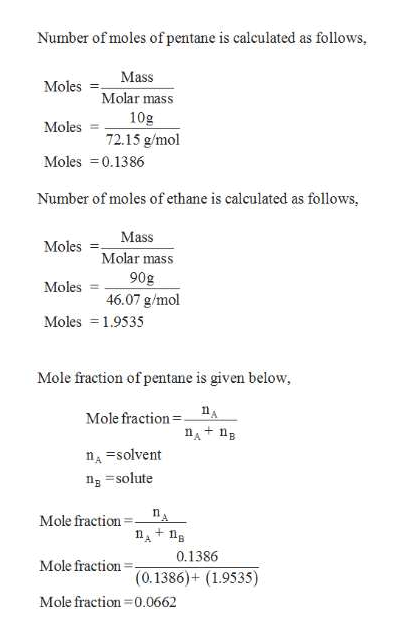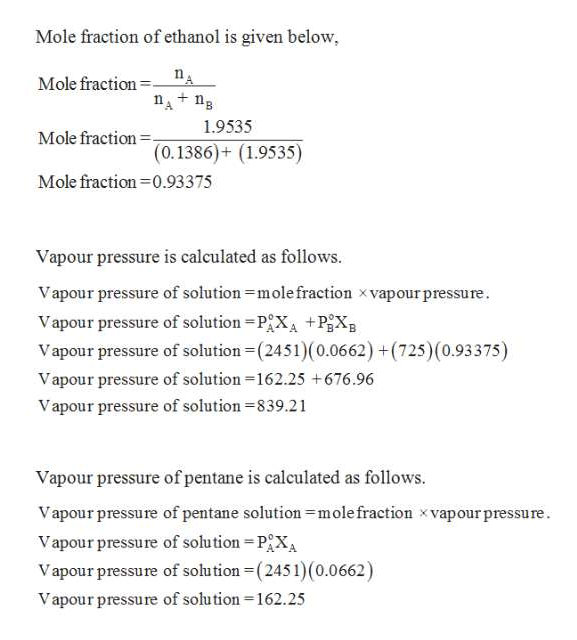Question

Calculate the percent by mass of pentane in the boiling solution here some day you may need in the picture round your answer to two significant digits

check_circleExpert Solution
Step 1

Given,

Vapour pressure of pentane = 2451. torr

Vapour pressure of ethanol = 725 torr.

Mass of pentane = 10 g

Mass of ethanol = 90 g

Molecular weight of pentane = 72.15 g/mol

Molecular weight of ethanol = 46.07 g/molhelp_outlineImage TranscriptioncloseNumber of moles of pentane is calculated as follows, Mass Moles Molar mass 10g Moles 72.15 g/mol Moles 0.1386 Number of moles of ethane is calculated as follows, Mass Moles Molar mass 90g 46.07 g/mol Moles Moles 1.9535 Mole fraction of pentane is given below Mole fraction A nA n nsolvent ng solute Mole fraction + 0.1386 Mole fraction (0.1386)+ (1.9535) Mole fraction 0.0662 fullscreen
Step 2

vapour pressure is ...help_outlineImage TranscriptioncloseMole fraction of ethanol is given below, Mole fraction nAn + 1.9535 Mole fraction (0.1386)+ (1.9535) Mole fraction 0.93375 Vapour pressure is calculated as follows Vapour pressure of solution mole fraction xvapour pressure. Vapour pressure of solution PX +PgX Vapour pressure of solution (2451)(0.0662) +(725) (0.93375) Vapour pressure of solution 162.25 +676.96 Vapour pressure of solution 839.21 Vapour pressure of pentane is calculated as follows. Vapour pressure of pentane solution mole fraction x vapour pressure. Vapour pressure of solution PXA Vapour pressure of solution (2451) (0.0662) Vapour pressure of solution -162.25 fullscreen

### Want to see the full answer?

See Solution

#### Want to see this answer and more?

Solutions are written by subject experts who are available 24/7. Questions are typically answered within 1 hour*

See Solution
*Response times may vary by subject and question
Tagged in

### General Chemistry